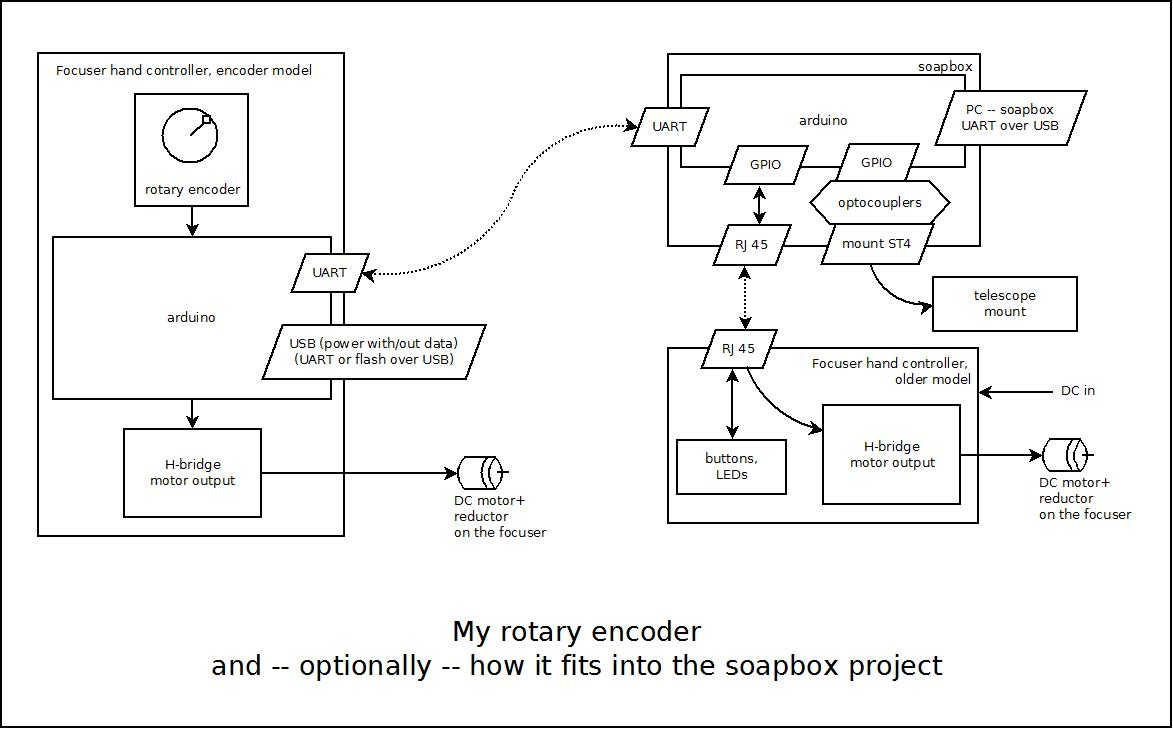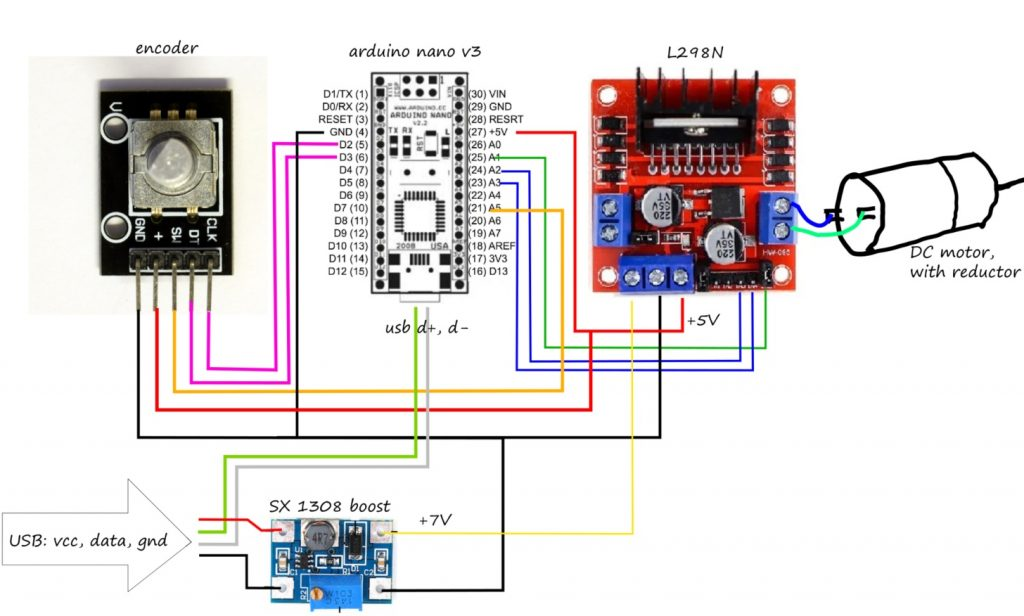So after I built a focuser controlled by a joystick, and considered it a usability failure, I built one based on an encoder, and modified the soapbox accordingly, maintaining backward compatibility. This encoder based hand controller has its own arduino, and connects to the soapbox, and through it to the PC to receive commands from the desktop app — but this connection is optional, it is fully functional as a standalone device. Below is the block diagram, explaining how it optionally fits into the soapbox daisy chain. Most variables are hand tuned for the DC motor + reductor gear box in use, as the source code reveals.The bare minimum wiring (for a given version of the code, may not coincide with the one published here)In the video I talk in Hungarian

```// a very basic encoder-based focuser, for the same motor,
//  sideproject
// in fact, the encoder is a very natural feel, somewhat opposed to the buttons

#include <Encoder.h>
#include <SoftwareSerial.h>
SoftwareSerial mySerial(8, 4); // RX, TX

#define PIN_ENABLE A1
#define PIN_LEFT A2
#define PIN_RIGHT A3
#define PIN_BUTTON A5
#define PIN_ENCODER_1 2
#define PIN_ENCODER_2 3

// Change these pin numbers to the pins connected to your encoder.
//   Best Performance: both pins have interrupt capability
//   Good Performance: only the first pin has interrupt capability
//   Low Performance:  neither pin has interrupt capability
Encoder knobLeft(PIN_ENCODER_1, PIN_ENCODER_2);
//   avoid using pins with LEDs attached

void setup() {
Serial.begin(57600);
Serial.println("Encoder Test:");

pinMode(PIN_ENABLE, OUTPUT);
pinMode( PIN_LEFT, OUTPUT);
pinMode( PIN_RIGHT, OUTPUT);

digitalWrite(PIN_ENABLE, LOW);
digitalWrite( PIN_LEFT, LOW);
digitalWrite( PIN_RIGHT, LOW);

pinMode( PIN_BUTTON, INPUT_PULLUP);

mySerial.begin(4800);

//moveRight(10000);

}

int timeRemainsLeft = 0;
int timeRemainsRight = 0;

void moveLeft(int duration){
timeRemainsLeft += duration;
timeRemainsRight = 0;
}

void moveRight(int duration){
timeRemainsRight += duration;
timeRemainsLeft = 0;
}

int old8 = 0; int old9 = 0; int old10 = 0;
void setPorts(int D, int E){
int new8 = D > 0 ? 1 : 0;
if (old8 != new8){
digitalWrite( PIN_LEFT, new8 == 1 ? HIGH : LOW);
old8 = new8;
}
int new9 = D < 0 ? 1 : 0;
if (old9 != new9){
digitalWrite( PIN_RIGHT, new9 == 1 ? HIGH : LOW);
old9 = new9;
}
int new10 = E;
if (old10 != new10){
digitalWrite( PIN_ENABLE, new10 == 1 ? HIGH : LOW);
old10 = new10;
}
}

int mpC = 0;
int kpC = 0;
void moveProcess(){
int f = 0;
int t = 0;
mpC++;
if (mpC > 16000){
mpC = 0;
}
if (timeRemainsRight > 0){
t = timeRemainsRight;
timeRemainsRight--;
f = 1;
}
if (timeRemainsLeft > 0){
int t = timeRemainsLeft;
timeRemainsLeft--;
f = -1;
}
if (f == 0){
setPorts(0,0);
}else{
int desired = (mpC % 4 != 0) ? 1 : 0;
if (t < 9){
desired = (mpC % 4 < 2) ? 1 : 0;
}
if (t < 5){
desired = (mpC % 4 == 0) ? 1 : 0;
}
if (t < 2){
desired = 1;
}

setPorts(f,desired);
}
}

long positionLeft  = 0;

unsigned long t0 = millis();
unsigned long t1 = t0;
unsigned long t3 = t0;
unsigned long t2;
unsigned long t6; int oldSign = 1;
unsigned long tOffset = 0;
unsigned long tOffsetStep = 1000000L;

inline void applyTimeOffset(){
if (t2 > 2*tOffsetStep){
t2 = t2 - tOffsetStep;
tOffset += tOffsetStep;
if (t1 > tOffsetStep){
t1 = t1 - tOffsetStep;
}
if (t0 > tOffsetStep){
t0 = t0 - tOffsetStep;
}
if (t3 > tOffsetStep){
t3 = t3 - tOffsetStep;
}
if (t6 > tOffsetStep){
t6 = t6 - tOffsetStep;
}

}
}

long nextCommand = 0;
unsigned long multiplier = 800;
unsigned long motorMultiplier = multiplier;
inline unsigned long motorMultiplierFunc(unsigned long m){
return (m * 7)/16 + 1;
}
unsigned long buttonLevel = 0;
unsigned long buttonLevelMultiplier = 8;
int backlash = 0;
int ignoreButton = 0;

#define FOCUS_COMMAND_START "foc="
#define SERIAL_TO_PORT_MULTI 2

inline void myserial_process(){
while (mySerial.available() > 0){
if (c == '#'){
}else{
if (c == '/'){
// process the daisy slave master commands
int handled = 0;
int len = strlen(FOCUS_COMMAND_START);
if (strncmp(mySerialReadBuffer, "foc=", len) == 0){
// please move the focuser left by amount that's after the M
}else{
}
}
}else{
}
}
};
}else{
//continue to concat
if (n < READ_BUFFER_LEN - 2) {
}
}
}
}
}

void loop() {
if (ignoreButton == 0){
mySerial.print("#focusbutton_push/");
ignoreButton = 50;
buttonLevel = (buttonLevel+1) % 2;
}
}
long newLeft;
t2 = micros() - tOffset;
applyTimeOffset();
if (t2 - t1 > 50*multiplier){
if (ignoreButton > 0){
ignoreButton--;
}
t1 = t2;
if (newLeft!=positionLeft) {
int sign = (newLeft - positionLeft) > 0 ? 1 : -1;
nextCommand += newLeft - positionLeft;
if (sign != oldSign){
backlash = 2;
if (t2 - t6 < 110*multiplier){
// noise
if (abs(nextCommand) < 5)
nextCommand = 0;
}
oldSign = sign;
}
t6 = t2;
positionLeft = newLeft;
}
if (t2 - t0 > 70*multiplier) if (nextCommand / 2 != 0){
t0 = t2;
Serial.print("Command = ");
Serial.print(nextCommand / 2);
Serial.println();
if (nextCommand < 0){
mySerial.print("#focusbutton_m/");
motorMultiplier = (multiplier*16) / 10;
moveRight(abs(nextCommand)*(3+buttonLevel*buttonLevelMultiplier)+1+backlash);
}else{
mySerial.print("#focusbutton_p/");
motorMultiplier = (multiplier*7) / 10;
moveLeft(abs(nextCommand)*(3+buttonLevel*buttonLevelMultiplier)+1+backlash);
}
backlash = 0;
nextCommand = 0;
}
}
if (t2 - t3 > motorMultiplierFunc(2*motorMultiplier)){
t3 = t2;
moveProcess();
}
myserial_process();
}```